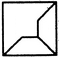# Non Verbal Reasoning - Shape Construction

Exercise : Shape Construction - Section 1
Directions to Solve

In each of the following questions, a set of five alternative figures 1, 2, 3, 4 and 5 followed by a set of four alternatives (A), (B), (C) and (D) is provided. It is required to select the alternative which represents three out of the five alternative figures which when fitted into each other would form a complete square.

1.

Select the alternative which represents three out of the five alternative figures which when fitted into each other would form a complete square.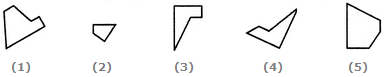145
245
123
234
Explanation: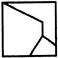2.

Select the alternative which represents three out of the five alternative figures which when fitted into each other would form a complete square.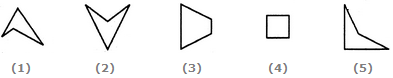124
345
123
135
Explanation: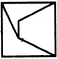3.

Select the alternative which represents three out of the five alternative figures which when fitted into each other would form a complete square.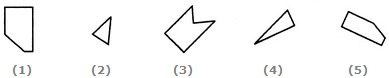124
125
234
245
Explanation: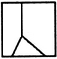4.

Select the alternative which represents three out of the five alternative figures which when fitted into each other would form a complete square.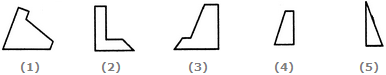123
124
135
145
Explanation: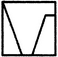5.

Select the alternative which represents three out of the five alternative figures which when fitted into each other would form a complete square.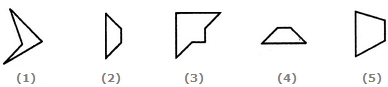134
345
234
135